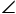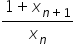# CAT 2021 Exam Question Papers Slot 1 | CAT Quants

### CAT Quantitative Aptitude | CAT 2021 Question Papers

CAT Question Papers: Solving CAT Question Papers from prior years will give CAT 2022 applicants invaluable insight into the examination format, question pattern, and level of difficulty. The CAT 2021 exams were held in three slots.

It is important for CAT preparation to examine old CAT Exam, Question Paper. In order to overcome the difficulties, they encounter when preparing for the CAT, aspirants must use a variety of strategies.

• By solving CAT Exam previous year question papers, aspirants learn about their own strengths and weaknesses. This allows them to plan their preparation accordingly.
• The secret to passing any exam is practice. Hence, by studying CAT exam papers over the last 5 to 10 years, you can attempt 500+ questions of all types and levels of difficulty.
• They can identify important topics by asking questions that have been asked repeatedly over time.
• CAT aspirants can benefit from numerous study materials and engaging video lessons available at Pathfinder For Me, including Free Download CAT Exam E-Books. Aspirants for the CAT test will get closer to passing the CAT 2022 exam with the help of these CAT question papers with solution PDF.

The best resource for CAT preparation is CAT question papers. Solving previous year’s CAT question papers helps candidates understand the CAT Exam Pattern, types of questions, question weightage, important topics, etc.

All CAT 2021 Quantitative aptitude questions have detailed answer keys and solutions provided by our experts. Watch the videos below to learn the fastest ways to answer the questions:

1).

The strength of an indigo solution in percentage is equal to the amount of indigo in grams per 100 cc of water. Two 800 cc bottles are filled with indigo solutions of strengths 33% and 17%, respectively. A part of the solution from the first bottle is thrown away and replaced by an equal volume of the solution from the second bottle. If the strength of the indigo solution in the first bottle has now changed to 21% then the volume, in cc, of the solution left in the second bottle is:

2).

Amar, Akbar and Anthony are working on a project. Working together Amar and Akbar can complete the project in 1 year, Akbar and Anthony can complete in 16 months, Anthony and Amar can complete in 2 years. If the person who is neither the fastest nor the slowest works alone, the time in months he will take to complete the project is

3).

The number of groups of three or more distinct numbers that can be chosen from 1, 2, 3, 4, 5, 6, 7 and 8 so that the groups always include 3 and 5, while 7 and 8 are never included together is

4).

A circle of diameter 8 inches is inscribed in the ∆ABC , whereABC =90o. If BC = 10 inches then the area of the triangle in square inches is

5).

Suppose hospital A admitted 21 less Covid infected patients than hospital B, and all eventually recovered. The sum of recovery days for patients in hospitals A and B were 200 and 152, respectively. If the average recovery days for patients admitted in hospital A was 3 more than the average in hospital B then the number admitted in hospital A was

6).

Suppose the length of each side of a regular hexagon ABCDEF is 2 cm . If T is the mid point of CD, then the length of AT in cm is

1. √15 cm

2. √13 cm

3. √12 cm

4. √14 cm

7).

The amount Neeta and Geeta together earn in a day equals what Sita alone earns in 6 days. The amount Sita and Neeta together earn in a day equals what Geeta alone earns in 2 days. The ratio of the daily earnings of the one who earns the most to that of the one who earns the least is

1. 7 : 3

2. 11 : 3

3. 11 : 7

4. 3:2

8).

The natural numbers are divided into groups as (1), (2, 3, 4), (5, 6, 7, 8, 9), ….. and so on. Then, the sum of the numbers in the 15th group is equal to

1. 6119

2. 7471

3. 4941

4. 6090

9).

Anu, Vinu and Manu can complete a work alone in 15 days, 12 days and 20 days, respectively. Vinu works everyday. Anu works only on alternate days starting from the first day while Manu works only on alternate days starting from the second day. Then, the number of days needed to complete the work is

1. 8

2. 6

3. 5

4. 7

10).

How many three-digit numbers are greater than 100 and increase by 198 when the three digits are arranged in the reverse order?

1. 80

2. 60

3. 50

4. 70

11).

Two trains cross each other in 14 seconds when running in opposite directions along parallel tracks. The faster train is 160 m long and crosses a lamp post in 12 seconds. If the speed of the other train is 6 km/hr less than the faster one, its length, in m, is

1. 184

2. 180

3. 190

4. 192

12).

If x0= 1 and x1 =2 and xn+2 =, n = 0, 1, 2, 3, 4, …, then the value of x2021 is equal to

1. 4

2. 3

3. 2

4. 1